# Basic Operators in Shell Scripting

There are 5 basic operators in bash/shell scripting:

• Arithmetic Operators
• Relational Operators
• Boolean Operators
• Bitwise Operators
• File Test Operators
1. Arithmetic Operators : These operators are used to perform normal arithmetics/mathematical operations. There are 7 arithmetic operators:
• Subtraction (-): Binary operation used to subtract two operands.
• Multiplication (*) :Binary operation used to multiply two operands.
• Division (/) :Binary operation used to divide two operands.
• Modulus (%) :Binary operation used to find remainder of two operands.
• Increment Operator (++) : Uniary operator used to increase the value of operand by one.
• Decrement Operator (–) : Uniary operator used to decrease the value of a operand by one

 `#!/bin/bash ` ` `  `#reading data from the user ` `read` `- p ``'Enter a : '` `a ` `           ``read` `    ``- p ``'Enter b : '` `b ` ` `  `          ``add ` `    ``= \$((a + b)) ` `        ``echo` `Addition of a and b are \$add ` ` `  `            ``sub ` `    ``= \$((a - b)) ` `        ``echo` `Subtraction of a and b are \$sub ` ` `  `            ``mul ` `    ``= \$((a * b)) ` `        ``echo` `Multiplication of a and b are \$mul ` ` `  `            ``div ` `    ``= \$((a / b)) ` `        ``echo` `division of a and b are \$div ` ` `  `            ``mod ` `    ``= \$((a % b)) ` `          ``echo` `Modulus of a ` `      ``and b are \$mod ` ` `  `      ``((++a)) ` `          ``echo` `Increment ` `          ``operator when applied on ``"a"` `results into a = \$a ` ` `  `      ``((--b)) ` `          ``echo` `Decrement ` `          ``operator when applied on ``"b"` `results into b = \$b `

Output: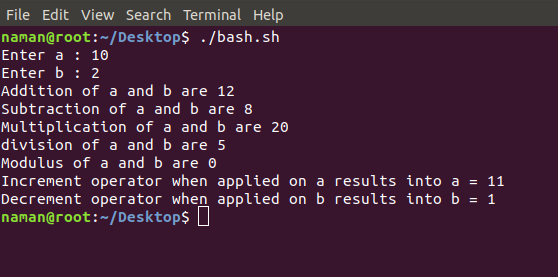2. Relational Operators : Relational operators are those operators which defines the relation between two operands. They give either true or false depending upon the relation. They are of 6 types:
• ‘==’ Operator : Double equal to operator compares the two operands. Its returns true is they are equal otherwise returns false.
• ‘!=’ Operator : Not Equal to operator return true if the two operands are not equal otherwise it returns false.
• ‘<' Operator : Less than operator returns true if first operand is lees than second operand otherwse returns false.
• ‘<=' Operator : Less than or equal to operator returns true if first operand is less than or equal to second operand otherwise returns false
• ‘>’ Operator : Greater than operator return true if the first operand is greater than the second operand otherwise return false.
• ‘>=’ Operator : Greater than or equal to operator returns true if first operand is greater than or equal to second operand otherwise returns false

 `#!/bin/bash ` `  `  `#reading data from the user ` `read` `-p ``'Enter a : '` `a ` `read` `-p ``'Enter b : '` `b ` `  `  `if``(( \$a==\$b )) ` `then` `    ``echo` `a is equal to b. ` `else` `    ``echo` `a is not equal to b. ` `fi` `  `  `if``(( \$a!=\$b )) ` `then` `    ``echo` `a is not equal to b. ` `else` `    ``echo` `a is equal to b. ` `fi` `  `  `if``(( \$a<\$b )) ` `then` `    ``echo` `a is ``less` `than b. ` `else` `    ``echo` `a is not ``less` `than b. ` `fi` `  `  `if``(( \$a<=\$b )) ` `then` `    ``echo` `a is ``less` `than or equal to b. ` `else` `    ``echo` `a is not ``less` `than or equal to b. ` `fi` `  `  `if``(( \$a>\$b )) ` `then` `    ``echo` `a is greater than b. ` `else` `    ``echo` `a is not greater than b. ` `fi` `  `  `if``(( \$a>=\$b )) ` `then` `    ``echo` `a is greater than or equal to b. ` `else` `    ``echo` `a is not greater than or equal to b. ` `fi`

Output: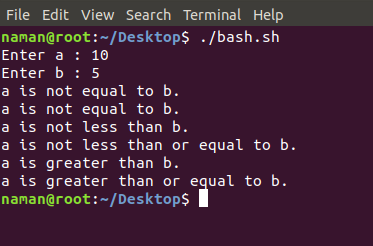3. Logical Operators : They are also known as boolean operators. These are used to perform logical operations. They are of 3 types:
• Logical AND (&&) : This is a binary operator, which returns true if both the operands are true otherwise returns false.
• Logical OR (||) : This is a binary operator, which returns true is either of the operand is true or both the operands are true and returns false if none of then is false.
• Not Equal to (!) : This is a uninary operator which returns true if the operand is false and returns false if the operand is true.

 `#!/bin/bash ` ` `  `#reading data from the user ` `read` `-p ``'Enter a : '` `a ` `read` `-p ``'Enter b : '` `b ` ` `  `if``((\$a == ``"true"` `& \$b == ``"true"` `)) ` `then` `    ``echo` `Both are ``true``. ` `else` `    ``echo` `Both are not ``true``. ` `fi` ` `  `if``((\$a == ``"true"` `|| \$b == ``"true"` `)) ` `then` `    ``echo` `Atleast one of them is ``true``. ` `else` `    ``echo` `None of them is ``true``. ` `fi` ` `  `if``(( ! \$a == ``"true"`  `)) ` `then` `    ``echo` `"a"` `was intially ``false``. ` `else` `     ``echo` `"a"` `was intially ``true``. ` ` ``fi`

Output: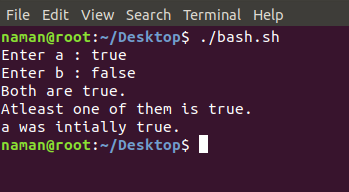4. Bitwise Operators : A bitwise operator is an operator used to perform bitwise operations on bit patterns. They are of 6 types:
• Bitwise And (&) : Bitwise & operator performs binary AND operation bit by bit on the operands.
• Bitwise OR (|) : Bitwise | operator performs binary OR operation bit by bit on the operands.
• Bitwise XOR (^) : Bitwise ^ operator performs binary XOR operation bit by bit on the operands.
• Bitwise compliment (~) : Bitwise ~ operator performs binary NOT operation bit by bit on the operand.
• Left Shift (<<) : This operator shifts the bits of the left operand to left by number of times specified by right operand.
• Right Shift (>>) : This operator shifts the bits of the left operand to right by number of times specified by right operand.

 `#!/bin/bash ` ` `  `#reading data from the user ` `read` `-p ``'Enter a : '` `a ` `read` `-p ``'Enter b : '` `b ` ` `  `bitwiseAND=\$(( a&b )) ` `echo` `Bitwise AND of a and b is \$bitwiseAND ` ` `  `bitwiseOR=\$(( a|b )) ` `echo` `Bitwise OR of a and b is \$bitwiseOR ` ` `  `bitwiseXOR=\$(( a^b )) ` `echo` `Bitwise XOR of a and b is \$bitwiseXOR ` ` `  `bitiwiseComplement=\$(( ~a )) ` `echo` `Bitwise Compliment of a is \$bitiwiseComplement ` ` `  `leftshift=\$(( a<<1 )) ` `echo` `Left Shift of a is \$leftshift ` ` `  `rightshift=\$(( b>>1 )) ` `echo` `Right Shift of b is \$rightshift `

Output: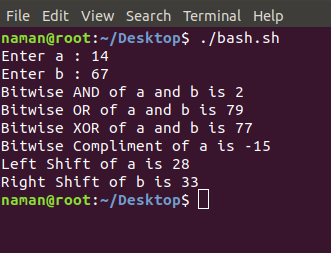5. File Test Operator : These operators are used to test a particular property of a file.
• -b operator : This operator check weather a file is a block special file or not. It returns true, if the file is a block special file otherwise false.
• -c operator : This operator checks weather a file is a character special file or not. It returns true if it is a character special file otherwise false.
• -d operator : This operator checks if the given directory exists or not. If it exits then operators returns true otherwise false.
• -e operator : This operator checks weather the given file exits or not. If it exits this operator returns true otherwise false.
• -r operator : This operator checks weather the given file has read access or not. If it has read access then it returns true otherwise false.
• -w operator : This operator check weather the given file has write access or not. If it has write then it returns true otherwise false.
• -x operator : This operator check weather the given file has execute access or not. If it has execute access then it returns true otherwise false.
• -s operator : This operator checks the size of the given file. If the size of given file is greater than 0 then it returns true otherwise it false.

 `#!/bin/bash ` ` `  `#reading data from the user ` `read -p ``'Enter file name : '` `FileName ` ` `  `if` `[ -e \$FileName ] ` `then ` `    ``echo File Exist ` `else` `    ``echo File doesnot exist ` `fi ` ` `  `if` `[ -s \$FileName ] ` `then ` `    ``echo The given file is not empty. ` `else` `    ``echo The given file is empty. ` `fi ` ` `  `if` `[ -r \$FileName ] ` `then ` `    ``echo The given file has read access. ` `else` `    ``echo The given file does not has read access. ` `fi ` ` `  `if` `[ -w \$FileName ] ` `then ` `    ``echo The given file has write access. ` `else` `    ``echo The given file does not has write access. ` `fi ` ` `  `if` `[ -x \$FileName ] ` `then ` `    ``echo The given file has execute access. ` `else` `    ``echo The given file does not has execute access. ` `fi `

Output: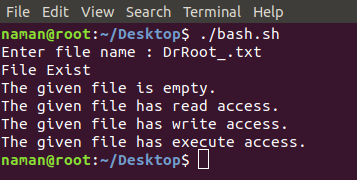My Personal Notes arrow_drop_upCheck out this Author's contributed articles.

If you like GeeksforGeeks and would like to contribute, you can also write an article using contribute.geeksforgeeks.org or mail your article to contribute@geeksforgeeks.org. See your article appearing on the GeeksforGeeks main page and help other Geeks.

Please Improve this article if you find anything incorrect by clicking on the "Improve Article" button below.

Improved By : ankitagarwal10